# Japan Meteorological Agency (‘JMA’) web page

‘JMA’ web page consists of some layers. You can use different function for download each component.

# Climate data

When you want to know how to prepare “data(climate_jp)”, please check url shown below.

https://github.com/matutosi/clidatajp/blob/main/data-raw/climate_jp.R

For polite scraping, 5 sec interval is set in download_climate(), it takes over 5 hours to get world climate data of all stations because of huge amount of data (3444 stations). Please use existing data by “data(climate_world)”, if you do not need to renew climate data.

  # existing data
data(climate_jp)
climate_jp %>%
dplyr::mutate_if(is.character, stringi::stri_unescape_unicode)

data(climate_world)
climate_world %>%
dplyr::mutate_if(is.character, stringi::stri_unescape_unicode)

# If you want links for all countries and all sations, remove head().
url <- "https://www.data.jma.go.jp/gmd/cpd/monitor/nrmlist/"
res <- gracefully_fail(url)
if(!is.null(res)){
\$("url")
climate <- list()
}
world_climate <- dplyr::bind_rows(climate)
world_climate
}

## Plot

Clean up data before drawing plot.

data(climate_world)
data(climate_jp)
climate <-
dplyr::bind_rows(climate_world, climate_jp) %>%
dplyr::mutate_if(is.character, stringi::stri_unescape_unicode)  %>%
dplyr::group_by(country, station) %>%
dplyr::filter(sum(is.na(temperature), is.na(precipitation)) == 0) %>%
dplyr::filter(period != "1991-2020" | is.na(period))

climate <-
climate %>%
dplyr::summarise(temp = mean(as.numeric(temperature)), prec = sum(as.numeric(precipitation))) %>%
dplyr::left_join(dplyr::distinct(dplyr::select(climate, station:altitude))) %>%
dplyr::left_join(tibble::tibble(NS = c("S", "N"), ns = c(-1, 1))) %>%
dplyr::left_join(tibble::tibble(WE = c("W", "E"), we = c(-1, 1))) %>%
dplyr::group_by(station) %>%
dplyr::mutate(lat = latitude * ns, lon = longitude * we)
#> summarise() has grouped output by 'country'. You can override using the
#> .groups argument.
#> Adding missing grouping variables: country
#> Joining with by = join_by(country, station)
#> Warning in dplyr::left_join(., dplyr::distinct(dplyr::select(climate, station:altitude))): Each row in x is expected to match at most 1 row in y.
#> ℹ Row 1 of x matches multiple rows.
#> ℹ If multiple matches are expected, set multiple = "all" to silence this
#>   warning.
#> Joining with by = join_by(NS)
#> Joining with by = join_by(WE)

Draw a world map with temperature.

climate %>%
ggplot2::ggplot(aes(lon, lat, colour = temp)) +
scale_colour_gradient2(low = "blue", mid = "gray", high = "red", midpoint = 15) +
geom_point() +
coord_fixed() +
theme_bw() +
theme(legend.key.size = unit(0.3, 'cm'))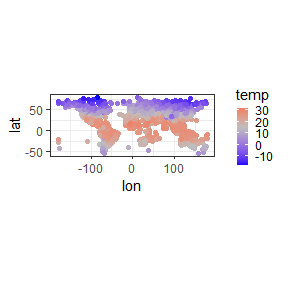# ggsave("temperature.png")

Draw a world map with precipitation except over 5000 mm/yr (to avoid extended legend).

climate %>%
dplyr::filter(prec < 5000) %>%
ggplot2::ggplot(aes(lon, lat, colour = prec)) +
scale_colour_gradient2(low = "yellow", mid = "gray", high = "blue", midpoint = 1500) +
geom_point() +
coord_fixed() +
theme_bw() +
theme(legend.key.size = unit(0.3, 'cm'))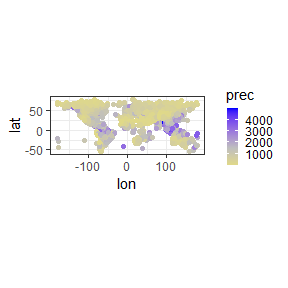# ggsave("precipitation.png")

Show relationships between temperature and precipitation except Japan.

japan <- stringi::stri_unescape_unicode("\\u65e5\\u672c")
climate %>%
dplyr::filter(country != japan) %>%
ggplot2::ggplot(aes(temp, prec)) +
geom_point() +
theme_bw() +
theme(legend.position="none")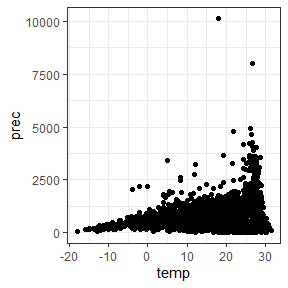# ggsave("climate_nojp.png")

Show relationships between temperature and precipitation including Japan.

climate %>%
ggplot2::ggplot(aes(temp, prec)) +
geom_point() +
theme_bw()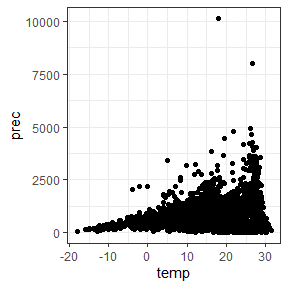# ggsave("climate_all.png")

Show relationships between temperature and precipitation. Blue: Japan, red: others.

climate %>%
dplyr::mutate(jp = (country == japan)) %>%
ggplot2::ggplot(aes(temp, prec, colour = jp)) +
geom_point() +
theme_bw() +
theme(legend.position="none")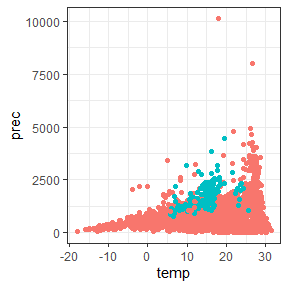# ggsave("climate_compare_jp.png")